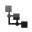#Summary

Rana Munns

### Definition

Growth models developed from populations of single cells can be extended mathematically to cover complex multicellular organisms where whole-plant growth is expressed in terms of leaf area and nutrient resources.

### Relative growth rate – Cell populations

A population of unicellular organisms presented with abundant resources and ample space will increase exponentially. Population doubling time Td (hours or days) is a function of an inherent capacity for cell division and enlargement which is influenced by environmental conditions, especially temperature.

Exponential curves are described mathematically as

N(t) = N0 ert (Eqn. 1)

where N(t) is the number of cells present at time t, N0 is the population at time 0, r describes the rate at which the population grows, and e is the base of natural logarithms.

By derivation from Eqn. 1

r = (1/N)(dN/dt) (Eqn. 2)

and is called relative growth rate with units of 1/time. Doubling time is Td=(ln 2)/r.

If cell growth data are converted to natural logarithms, a linear increase over time will be apparent. For strict exponential growth where N(t) is given by Eqn. 1,

ln N(t) = ln N0 + rt (Eqn. 3)

so that a plot of ln N(t) as a function of time t is a straight line whose slope is relative growth rate r.

In practice, r is estimated by assessing cell numbers N1 and N2 on two occasions, t1 and t2 (separated by hours or days depending on doubling time – most commonly days in plant cell cultures), and substituting those values into the expression

r = (ln N2 – ln N1) / (t2t1) (Eqn. 4)

which expresses r in terms of population numbers N1 and N2 at times t1 and t2, respectively.

If growth is not exponential, relative growth rate r is not constant, but the concept of relative growth rate is still useful for analysis of growth dynamics in populations or organisms.

### Relative growth rate – Plant biomass

As cell number is an impractical measure of growth in whole plants, biomass (W) is generally taken as a surrogate for carbon gain and referenced to the number of days elapsed between successive observations. At any instant, relative growth rate, RGR (d-1), can be expressed in terms of differential calculus as RGR = (1/W)(dW/dt) (compare Eqn. 2) so that RGR is increment in dry mass (dW) per increment in time (dt) divided by existing biomass (W). Averaged over a time interval t1 to t2 during which time biomass increases from W1 to W2, RGR (d-1) can be calculated from

RGR = (ln W2 – ln W1) / (t2t1) (Eqn. 5)

Values of RGR for a fast-growing plant are about 0.25 d-1. The RGR is highest when the plant is smallest, and there is no shading of leaves (see Fig. 1 below). It falls as the plant grows larger because each leaf does not receive the optimal amount of light. It is lower when there are other resource limitations such as nitrogen and water.

RGR (biomass increase over time per unit of starting biomass over time) can be a much more useful measure of growth than just biomass increase over time, as RGR takes into account the starting biomass, that is, the size of the plant at the start of the experimental period.

### Functional growth analysis

RGR is made up of two components, the leaf area ratio (LAR) and the net assimilation rate (NAR). LAR is the leaf area per unit total plant mass, and the NAR is the rate of increase in plant mass per unit leaf area.

RGR = LAR x NAR

Each of these terms can be divided into measurable quantities that influence the growth rate. LAR is the product of specific leaf area (SLA) and the leaf mass ratio (LMR). SLA is the leaf area per unit leaf mass, and LMR is the fraction of the total plant biomass allocated to leaves.

LAR = SLA x LMR

NAR is the net result of the rate of carbon gain in photosynthesis per unit leaf area, and its use in respiration of leaves, stems and roots.

Further description of growth components are given in Chapter 6 of Plants in Action (Atwell et al. 1999) and Chapter 7 of Plant Physiological Ecology (Lambers et al. 2008)Fig. 1. RGR for whole plants decreases as size increases. The curve shown is derived from data for three genotypes of a semi-dwarf wheat and designated by three different symbols. The three genotypes differ in their complement of dwarfing genes, and thus in final size and absolute growth rate, but when referrenced to plant mass there are no intrinsic differences in RGR. Figure reproduced from Plants in Action (Eds. Atwell et al., 1999)Figure 6.20, with permission.

### Literature references

Chapter 6 in Plants in Action: http://plantsinaction.science.uq.edu.au/edition1/

Lambers, H., F.S. Chapin III, T.L.Pons. 2008 (2nd Edition). Chapter 7 in Plant Physiological Ecology. Springer, New York.